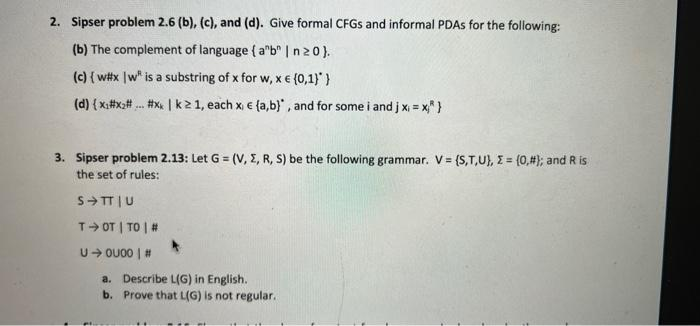# Question 2. Sipser problem 2.6 (b), (c), and (d). Give formal CFGs and informal PDAs for the following: (b) The complement of language { ab | n20). (c) { w#xw" is a substring of x for w, x € (0,1)) (d) { x#x#... #xxlk21, each x € (a,b), and for some i and j < = x } 3. Sipser problem 2.13: Let G = (V, E, R, S) be the following grammar. V = {S,T,U, I = (0,#}; and Ris the set of rules: STTU TOT TO # U OUOO # a. Describe L(G) in English. b. Prove that L(G) is not regular.GISISS The Asker · Computer ScienceTranscribed Image Text: 2. Sipser problem 2.6 (b), (c), and (d). Give formal CFGs and informal PDAs for the following: (b) The complement of language { ab | n20). (c) { w#xw" is a substring of x for w, x € (0,1)) (d) { x#x#... #xxlk21, each x € (a,b), and for some i and j < = x } 3. Sipser problem 2.13: Let G = (V, E, R, S) be the following grammar. V = {S,T,U, I = (0,#}; and Ris the set of rules: STTU TOT TO # U OUOO # a. Describe L(G) in English. b. Prove that L(G) is not regular.
More
Transcribed Image Text: 2. Sipser problem 2.6 (b), (c), and (d). Give formal CFGs and informal PDAs for the following: (b) The complement of language { ab | n20). (c) { w#xw" is a substring of x for w, x € (0,1)) (d) { x#x#... #xxlk21, each x € (a,b), and for some i and j < = x } 3. Sipser problem 2.13: Let G = (V, E, R, S) be the following grammar. V = {S,T,U, I = (0,#}; and Ris the set of rules: STTU TOT TO # U OUOO # a. Describe L(G) in English. b. Prove that L(G) is not regular.